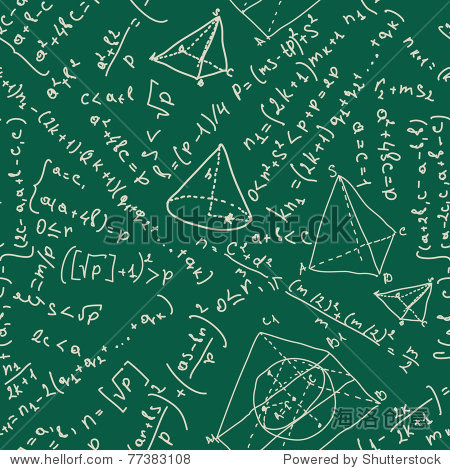cyclic group

## 性质

(1)若|a|=∞，则(a)与整数加群Z同构；
(2)若IaI=n，则(a)与模n的剩余类加群Zn同构。
(1)|a|=∞，则当m≠n时，
am≠an，(a)={…，a-2，a-1，e，a1，a2，…}.

φ(am·an)=φ(am+n)=m+n=φ(am)+φ(an)，

(2)设IaI=n，则(a)={e，a，a2，…，an-1}

σ(am·am')=σ(am+m')
=[m+m′]=[m]+[m′]
=σ(am)+σ(am′)，

(1)|[k]|=n/(k,n)
(2)[k]是Zn的生成元<=>(k，n)=1.
(1)由定理可得.
(2)若[k]∈Zn，则([k])⊆Zn，由(1)与(k，n)=1知|[k]|=n，所以|([k])|=n，Zn=([k])

## 词条相册